# Introduction

Outperforming the state-of-the-art BERT algorithm on 20 Natural Language Processing(NLP) benchmark tasks, XL-Net is a recent advancement in the field of NLP developed by Google and Carnegie Mellon University.  Considered as one of the 2019’s most important developments in NLP, XL-Net combines the autoregressive language model, Transformer-XL, and bidirectional capability of autoencoders, BERT, to unleash the power of this important language modeling tool.

With the advancement of NLP tools in the tasks like machine translation, sentiment analysis, reading comprehension, summarization and question answering, there have been many successful developments of unsupervised representation learning methods such as BERT, ELMo, CoVe. These methods first pretrain neural networks on a large-scale unlabeled text corpora, and then fine-tune the models on task specific datasets such as question answering, sentiment analysis, natural language inference, document ranking and so on.

XL-Net combines the best of both the AutoRegressive language model and AutoEncoders, the two most well-known pre-training objectives, while avoiding their limitations, to achieve the state-of-the-art result. Before exploring the details of this new algorithm, let’s first dive into some concepts and previous works that led to its discovery.

# Language Model

Language model computes the probability of a sentence( sequence of words) or the probability of the next word in a sequence. For eg: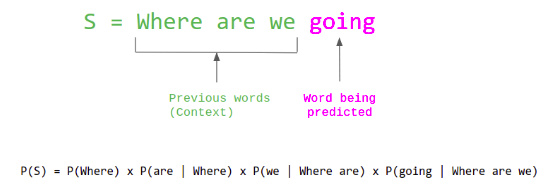The maximum likelihood objective decomposes into a sequence of prediction problems for each term(word) in the sequence given the previous terms.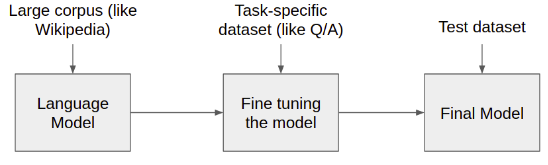Pre-training a language model on a large corpus to predict the next token in a sequence, the learned weights can be used as inputs to separate fine tuning models for other downstream tasks in NLP. The fine tuned model can now perform a specific task which is again tested with a different dataset to obtain a final model.

The two such most common language models are : Autoregressive Language Models and Autoencoders.

# AutoRegressive Language Model

Autoregressive model predicts the value for the next time step($t$+$1$) given the observations of the last time steps ($0$,…,$t$) as inputs to a regression equation:

X(t+1) = b0 + b1X(t-1) + b2X(t-2)

The name autoregression comes from the fact that the regression model uses the data from the same input variable just at different time steps. In NLP, the autoregressive language model estimates the probability distribution of a text corpus with an autoregressive model meaning.

For example Given a text sequence x = (x1, · · · , xT ), AR language modeling factorizes the likelihood into a forward product :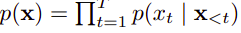Or a backward product: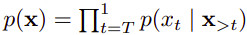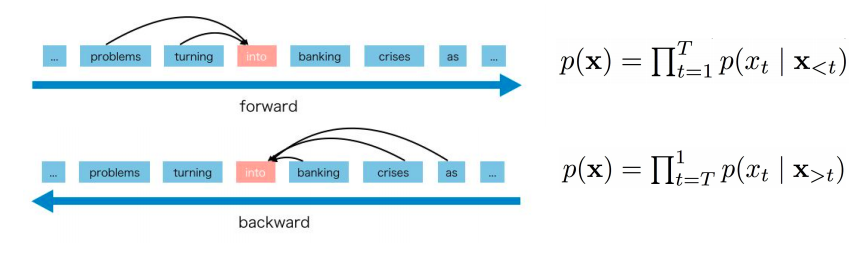## Limitations of AR Language Model

AR language models only encode the unidirectional context either in the forward or backward direction in a text sequence. This leads to an inability to capture bidirectional context which is often required for downstream language understanding tasks.

# AutoEncoders

Auto-encoder tries to reconstruct the original input from the encoded/corrupted data. An example of this model is BERT, a state-of-the-art language model in which given the input token sequence, a certain portion of tokens are replaced by a special symbol [MASK], and the model is trained to recover the original tokens from the corrupted version.

For example :

Given an original sentence “The cat sat on the wall”. The input to BERT would be “The cat [MASK] on the [MASK]” where the model tries to reconstruct the original sentence by predicting the masked tokens.

It uses bidirectional contexts and thus leads to better performance than AR language modelling.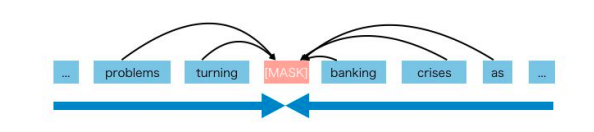## Limitations of Autoencoders

• Pre-train finetune discrepancy: The artificial tokens [Mask] appear during pre-training only and not during fine-tuning which creates a discrepancy between pretraining and fine tuning.
• Independent prediction: As BERT assumes the predicted tokens are independent of each other, given unmasked tokens it fails to capture the relationship among the predictions. For eg: For a sequence (New, York, is, a, city) where ([Mask],[Mask], is, a, city) being an input to the BERT, the prediction for both the tokens ‘New’ and ‘York’ is dependent on (is, a, city) and is not dependent on each other. This means that the ‘York’ token for the second mask is as probable as ‘Angeles’(of Los Angeles) in the given sequence without the knowledge of the prediction of the first token.

We see that both the AR language model and AE have their own limitations while also having some major advantages over each other. Therefore, XL-Net was proposed to overcome these limitations and utilize the benefits of AR language model while having it learn from bidirectional context as AE models (e.g., BERT) during the pre-training phase.

# Transformer

Transformer is based on attention mechanism where instead of processing tokens one by one as Autoregressive model does, it captures the relationship between all the tokens in a segment at once using three learned weight matrices; Query, Key and Value forming an attention head. Along with attention, it also introduces positional encoding to help the network learn the position of each token within the segment when they are processed parallely.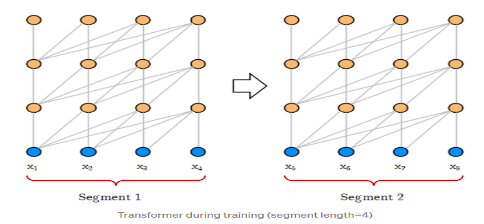Image:source

BERT is based on the transformer model and hence, makes use of the attention mechanism. If you’re not familiar with the attention mechanism and the architecture of transformer, I would recommend you to go through this descriptive blog. illustrated-transformer.

## Limitations of Transformer

1. Limited context-dependency: As the model is trained on segments, it can learn only from the tokens within the same segments and is independent of the tokens that appeared in other segments. The maximum dependency distance between characters is limited by the segment length.
2. Context Fragmentation: Fixed-length segments that exceed the upper bound of segment length are trained separately from scratch which leads to context fragmentation.

For example: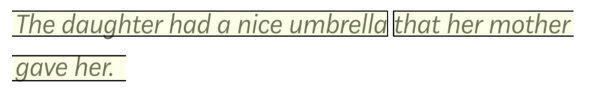Image:source

If the sentence length is higher than the segment length and is fragmented into two segments then there is no dependency or relationship between the two segments of the same sentence hence, the learned vector for the word “her” won’t be able to incorporate the information from the word “daughter” making it difficult for the model to predict the next word to be “mother”.

To overcome these two limitations of the transformer, Transformer-XL makes use of two techniques: segment level recurrence mechanism and relative positional encoding.

# Transformer-XL

Transformer-XL captures longer dependencies than RNN and Transformer with the help of segment level recurrence mechanism and relative positional encoding.

## Segment Level Recurrence Mechanism

The Segment Level Recurrence Mechanism addresses context fragmentation and enables long-term dependencies by using information from previous segments. Transformer-XL caches the previous hidden states of the previous segment and concatenates to the previous hidden states of the current segment to extend the context for when the model processes the next hidden state of the same segment. Suppose we have two segments x̃ and x of length T each, with permutations z̃ and z respectively. We process the first segment according to z̃ and cache the hidden states h̃ for every layer. For segment x, we can write the update equation as: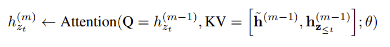where, h˜(m) is the hidden state of the previous segment for layer m and [., .] denotes concatenation along the sequence dimension. This allows sentence segments from the past to be reused with the new segment.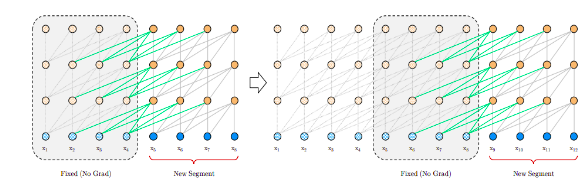Image:source

Caching of the hidden states is not only limited to a previous segment but can also be done for several previous segments in the same way to incorporate longer dependencies but ofcourse, under the limitations of the GPU memory.

## Relative Positional Encodings

The absolute positional encoding in Transformer handles each segment separately which leads to tokens from different segments having the same positional encoding. For example, the first token of the first and the second segments will have the same encoding, although their position and importance across segments are different. To overcome this, Transformer-XL uses relative positional encoding which instead of just adding a fixed positional encoding to the word embedding only before the first layer(as in Transformer), dynamically adds the encoding to each attention module and is based on the relative distance between tokens(Query and Keys).

It adds the following three changes in the simple multiplication of the Attention Head’s Score ($Q$i⋅$K$j), where $i$ refers to rows containing queries and $j$ refers to columns containing keys:

1. Replaces absolute positional encoding(P$j$) with the relative positional encoding(P$i$-$j$) where, $i$-$j$ refers to the distance between the query and the key.
2. Replaces the query term with a position-independent trainable parameter.
3. Separates the weight vectors for producing the content-based and location-based key vectors.

You can find the detailed explanation of these changes in the Transformer-XL blog.

# Permutation Language Modelling

XL-Net uses a variant of language modelling called ‘Permutation Language modelling’ which allows AR models to capture bidirectional context. Consider a sequence x with $N$ number of tokens. Then, there are $N!$ different ways to do valid autoregressive factorization. In each permutation/factorization order, the ($t$-1) tokens that proceed the target token (at $t$-th position) will be fed forward into the hidden layers to predict the $t$-th token. Training the model on many such permutations of the factorization order in an autoregressive way, the model learns to capture the information from both sides and learns the dependencies between all combinations of inputs. It avoids the pretrain-finetune discrepancy and the independence assumption of BERT being based on AR framework.

Note: We do not permute the input sequence but only permute the factorization order as input sequence follows a natural order during fine-tuning.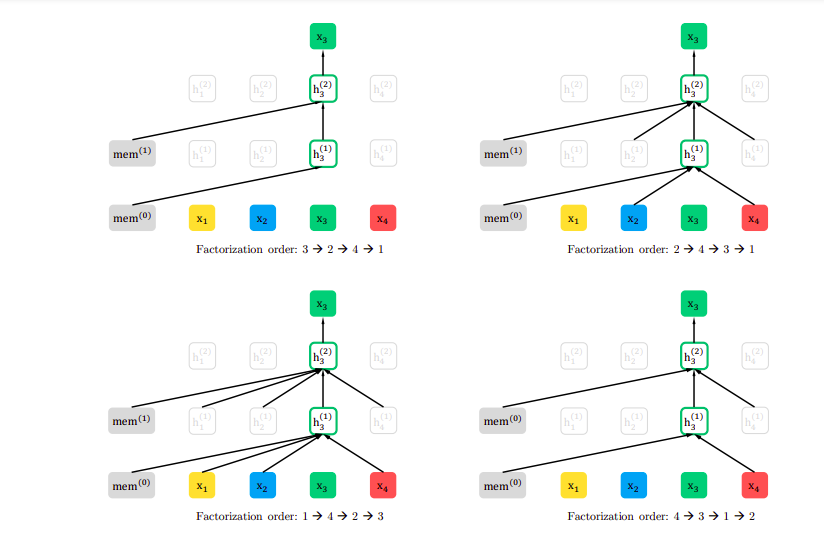Image:source

The above illustration shows 4 different factorization orders for predicting the x3 token given the same input sequence x1 →x2 →x3 →x4 with 4 tokens.

The objective function of the model is to maximize the log-likelihood of a sequence w.r.t all possible permutations of the factorization order: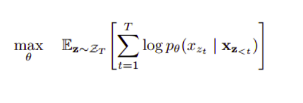where Zₜ is the set of all possible permutations of length t, zₜ denotes t-th element and z_<t denotes first $t-1$ elements of the permutation z ∈ Zₜ. So, it uses the first ($t-1$) tokens in the factorization order to predict the $t$-th token.

# Target Aware Two-Stream Self-Attention ArchitectureImage:source

Here, we can see that two different permutations z1(4->1->2->5->3) and z2(4->1->2->5->3) have the same context i.e 4->1->2 and if we were to predict the word in fourth position then the function gives the same prediction for two different target position. So, we take the context and the target position and re-parameterise the next-token distribution to be target position aware as shown below: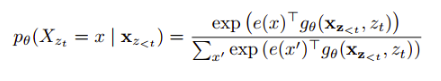where,is a new type of hidden representation that takes both context as well as position of the target ,z_t, as inputs. The standard Transformer contradicts the following two requirements for $g$:

• To predict token at position zₜ, g should only use the position zₜ and not the content at zₜ. Otherwise, the objective becomes trivial.
• To predict tokens at positions greater than zₜ, $g$ should also encode content at the current position to provide full contextual information.

This conflict is resolved by using two sets of hidden representation instead of just one:

• The content representation hθ(x_z_≤t): It serves a similar role to the standard hidden states in Transformer which encodes both the context and xzt itself.
• The query representation gθ(x_z_<t , z_t): This representation only has access to the contextual information x_z_<t and the position z_t, but not the content x_z_t.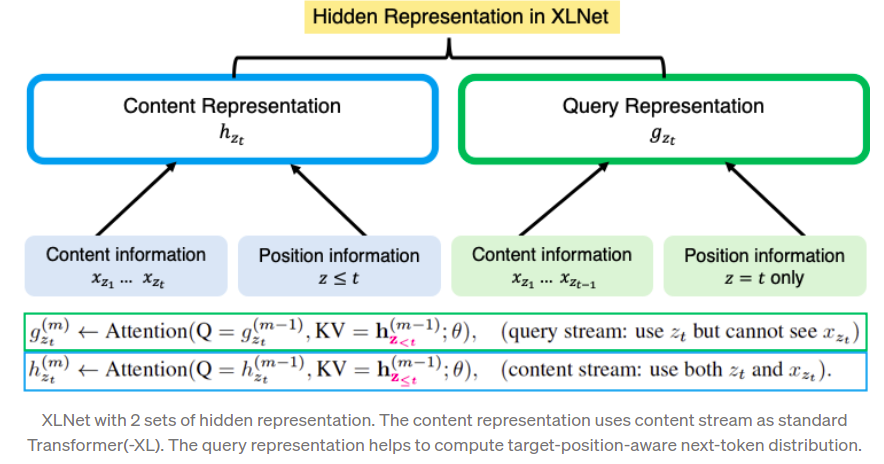Image:source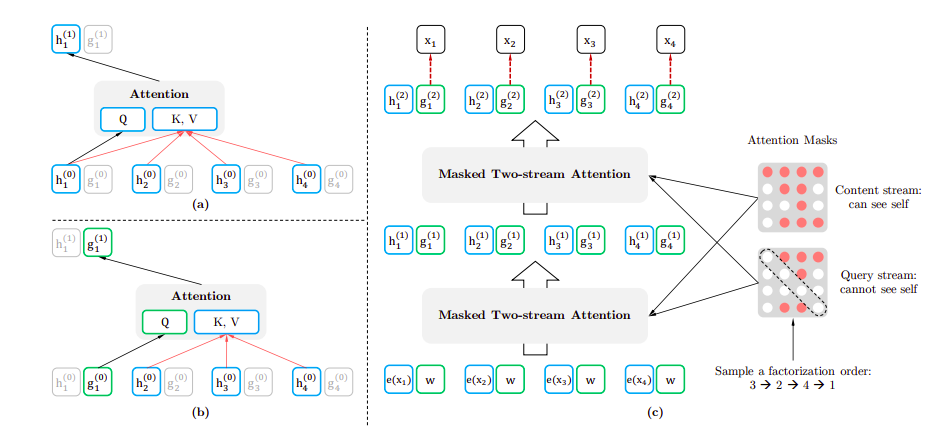Image:source

The first layer content stream is initialized to the corresponding word embedding, i.e: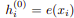and the query stream is initialized to the trainable vector, $w$. For each self-attention layer $m$ = 1, . . . , $M$, the two streams are updated as follows: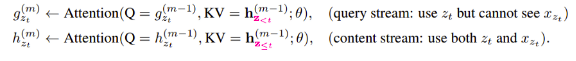where $Q$, $K$ and $V$ denote query, key and value respectively. Then, the query representation($g$) from the last layer is used in the proposed objective of language modelling.

# Partial Prediction

Instead of considering all the tokens in a permutation which leads to a difficult optimization problem and slower convergence, we only predict the last tokens in a factorization order of a sequence. So, we split z into a non-target subsequence z ≤ c and a target subsequence z > c , where c is the cutting point.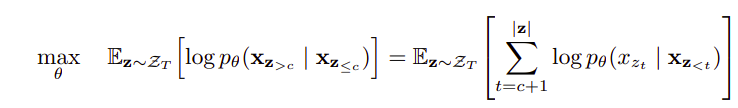Here, the objective function maximizes the log-likelihood of the target subsequence conditioned on the non-target subsequence.

# Modeling Multiple Segments

Some of the downstream tasks have multiple input segments like a question and a context paragraph in question answering. During pre-training, similar to Bert, we take two sentence segments- A and B where 50% of the time A is followed by B in the corpus and 50% of the time B is a random sentence. We then concatenate the two segments A and B as one sequence and apply permutation language modelling. Here, we reuse the hidden states of A only if B followed A. The input to the model is same as that of BERT- [A, SEP, B, SEP, CLS], where SEP and CLS are special symbols and A and B are sentence segments.

# Relative Positional Encodings

Instead of adding absolute segment embedding to the word embedding at each position of the segment to distinguish between sentence segments as in BERT, we use relative segment encodings. Given a pair of positions $i$ and $j$, if they are from the same segment we can use segment encoding $s⁺$ else $s⁻$, where $s⁺$ and $s⁻$ are learnable model parameters. When $i$ attends to $j$, we compute $s$ (equal to $s⁺$ or $s⁻$ depending on whether $i$ and $j$ belong to the same segment or not). Then, we compute attention weight by taking the dot product of query $qᵢ$ and $s$ and add this weight to the standard self-attention weight. This makes it possible to finetune on tasks having more than two segments.

# Implementation Detail of XL-Net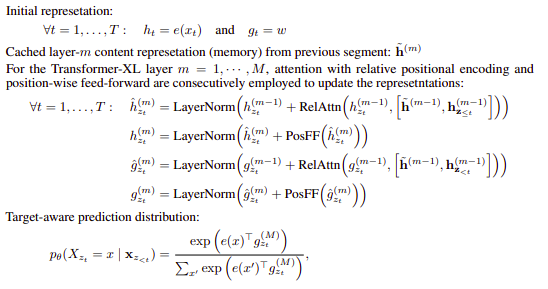Image:source

We can see that the implementation also includes multi-head attention, residual connections, layer normalization and position-wise feed-forward network as does the standard transformer architecture.

# Hyperparameters

For pre training: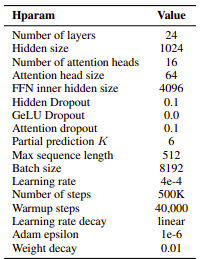Image:source

For Fine tuning: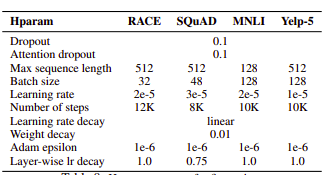Image:source

# Experiments conducted with XLNET

XL-Net performance was significantly larger for explicit reasoning tasks like SQuAD and RACE that involve longer context requirements. Ablation study performed on XLNet shows that Transformer-XL backbone and the permutation LM play a heavy role in improving XLNet’s performance over that of BERT.

Following are the datasets XLNET was tested by the authors of the paper.

• RACE (ReAding Comprehension from Examinations)— 100K questions from English exams, a challenging benchmark for long text understanding, XLNET outperforms the best model by 7.6 points in accuracy.